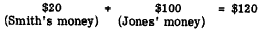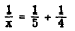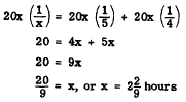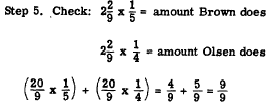General form of a linear equationCustom SearchGENERAL FORM OF A LINEAR EQUATION The expression GENERAL FORM, in mathematics, implies a form to which all expressions or equations of a certain type can be reduced. The only possible terms in a linear equation in one variable are the first-degree term and the constant term. Therefore, the general form of a linear equation in one variable is ax + b = 0 By selecting various values for a and b, this form can represent any linear equation in one variable after such an equation has been simpli represents the numerical equation     7x + 5 = 0 If a = 2m - n and b = p - q, then ax + b =0 represents the literal equation     (2m-n)x + p - q = 0 This equation is solved as follows:USING EQUATIONS TO SOLVE PROBLEMS To solve a problem, we first translate the numerical sense of the problem into an equation. To see how this is accomplished, consider the following examples and their solutions. EXAMPLE 1: Together Smith and Jones have \$120. Jones has 5 times as much as Smith. How much has Smith? SOLUTION: Step 1. Get the problem clearly in mind. There are two parts to each problem-what is given (the facts) and what we want to know (the question). In this problem. we know that Jones has 5 times as much as Smith and together they have \$120. We want to know how much Smith has. Step 2. Express the unknown as a letter. Usually we express the unknown or number we know the least about as a letter (conventionally we use x). Here we know the least about Smith�s money. Let x represent the number of dollars Smith has. Step 3. Express the other facts in terms of the unknown. If x is the number of dollars Smith has and Jones has 5 times as much, then 5x is the number of dollars Jones has. Step 4. Express the facts as an equation. The problem will express or imply a relation between the expressions in steps 2 and 3. Smith�s dollars plus Jones� dollars equal \$120. Translating this statement into algebraic symbols, we have     x + 5x = 120 Solving the equation for x,         6x = 120           x = 20 Thus Smith has \$20. Step 5. Check: See if the solution satisfies the original statement of the problem. Smith and Jones have \$120.EXAMPLE 2: Brown can do a piece of work in 5 hr. If Olsen can do it in 4 hr how long will it take them to do the work together? SOLUTION: Step 1. Given: Brown could do 5 hr. Olsen could do it in 4 hours. the work in Unknown: How long it takes them to do the work together. Step 2. Let x represent the time it takes them to do the work together. Step 3. Then 1/x is the amount they do together in 1 hr. Also, in 1 hour Brown does 1/5 of the work and Olsen does 1/4 of the work Step 4. The amount done in 1 hr is equal to the part of the work done by Brown in 1 hr plus that done by Olsen in 1 hr.Solving the equation,They complete the work together in 2 2/9: hours.Practice problems. Use a linear equation in one variable to solve each of the following problems: 1. Find three numbers such that the second is twice the first and the third is three times as large as the first. Their sum is 180. 2. A seaman drew \$75.00 pay in dollar bills and five-dollar bills. The number of dollar bills was three more than the number of five- draw? (Hint: If x is the number of five-dollar bills, then 5x is the number of dollars they represent.) 3. Airman A can complete a maintenance task in 4 hr. Airman B requires only 3 hr to do the same work. If they work together, how long should it take them to complete the job? Answers: 1. First number is 30. Second number is 60. Third number is 90. 2. Number of five-dollar bills is 12. Number of one-dollar bills is 15.Integrated Publishing, Inc. - A (SDVOSB) Service Disabled Veteran Owned Small Business